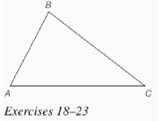Chapter 7.CR, Problem 18CRElementary Geometry For College St...

7th Edition
Alexander + 2 others
ISBN: 9781337614085

Solutions

Chapter
SectionElementary Geometry For College St...

7th Edition
Alexander + 2 others
ISBN: 9781337614085
Textbook Problem

In Review Exercises 18 to 23, use construction methods with the accompanying figure. Given: Δ A B CFind: The incenter

To determine

To find: The incenter of the triangle ABC.

Explanation

Given:

The triangle ABC is shown below.

Theorem used:

The three angle bisectors of the angles of a triangle are concurrent.

Calculation:

Consider the triangle ABC.

First draw the angular bisectors of A,B,C.

Still sussing out bartleby?

Check out a sample textbook solution.

See a sample solution

The Solution to Your Study Problems

Bartleby provides explanations to thousands of textbook problems written by our experts, many with advanced degrees!

Get Started

Limits at Infinity Find the limit. 18. limxsin2x

Precalculus: Mathematics for Calculus (Standalone Book)

In Exercises 7-10, find the slope of the line shown in each figure. 8.

Applied Calculus for the Managerial, Life, and Social Sciences: A Brief Approach

In Problems 1-4, find the differential of each function.

Mathematical Applications for the Management, Life, and Social Sciences

In words, explain what is measured by SS, variance, and standard deviation.

Statistics for The Behavioral Sciences (MindTap Course List)

Sometimes, Always, or Never: If for all n and both {an} and {cn} converge, then{bn} converges.

Study Guide for Stewart's Single Variable Calculus: Early Transcendentals, 8th

Minimize C=4x+y Subjected to 2x+y102x+3y24x+3y15x0,y0

Finite Mathematics for the Managerial, Life, and Social Sciences